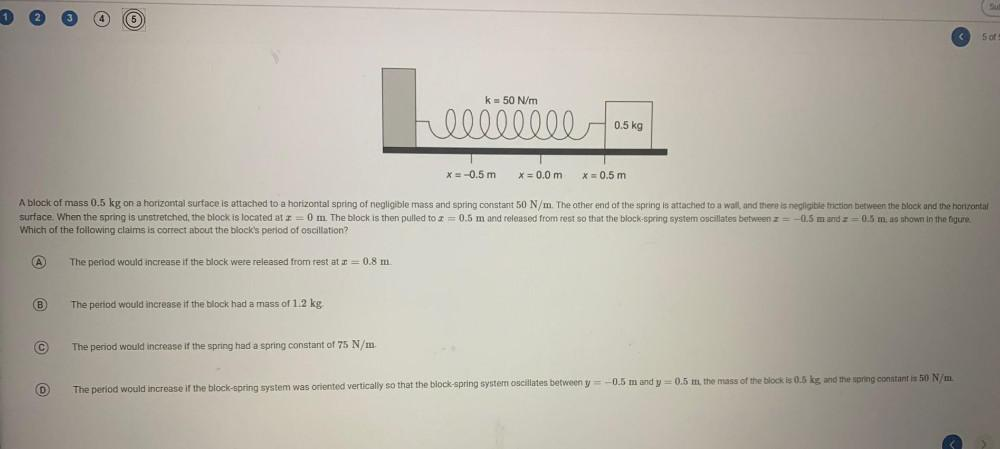Question:

# SU < 5 of k= 50 N/m heee edibilla 0.5 kg x = -0.5 m X = 0.0 m X = 0.5 m A block of mass 0.5 kg on a horizontal surface is attachSU < 5 of k= 50 N/m heee edibilla 0.5 kg x = -0.5 m X = 0.0 m X = 0.5 m A block of mass 0.5 kg on a horizontal surface is attached to a horizontal spring of negligible mass and spring constant 50 N/m. The other end of the spring is attached and there is negligible ction between the block and the horizontal surface. When the spring is unstretched, the block is located at 2=0 m. The block is then pulled to x = 0.5 m and released from rest so that the block-spring system oscillates between I = -0.5 m and I = 0.5 m, as shown in the figure. Which of the following claims is correct about the block's period of oscillation? A The period would increase if the block were released from rest at = 0.8 m. B The period would increase if the block had a mass of 1.2 kg. The period would increase if the spring had a spring constant of 75 N/m. D The period would increase if the block-spring system was oriented vertically so that the block-spring system oscillates between y = -0.5 m and y = 0.5 m, the mass of the block is 0.5 kg, and the spring constant is 50 N/m.